Finding determinant of a 2x2 matrix

Chapter 4 Class 12 Determinants
Concept wise

For a 2 × 2 matrix, like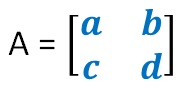Determinant is calculated like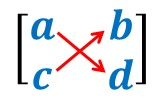So,

Let’s take an example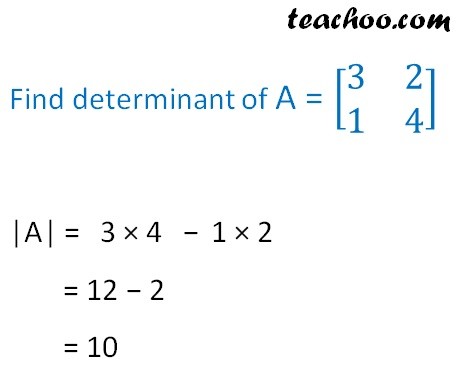## For a 3 × 3 matrix, like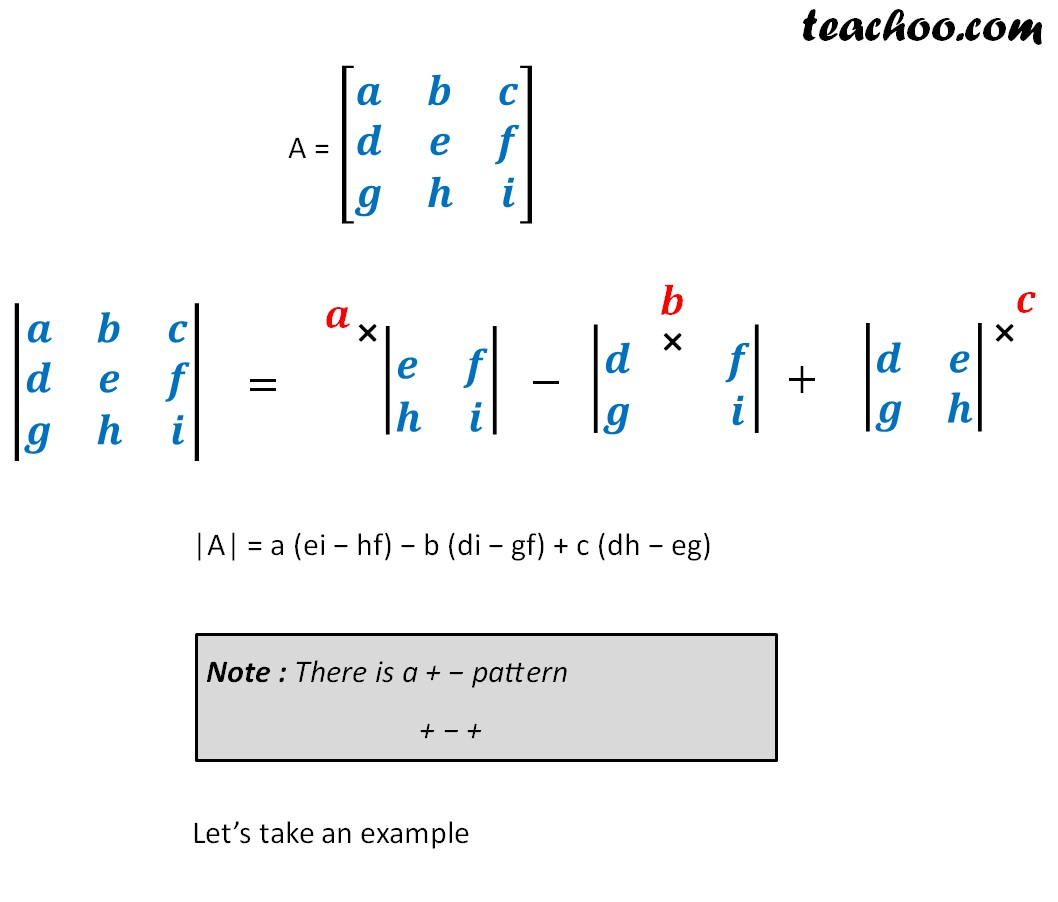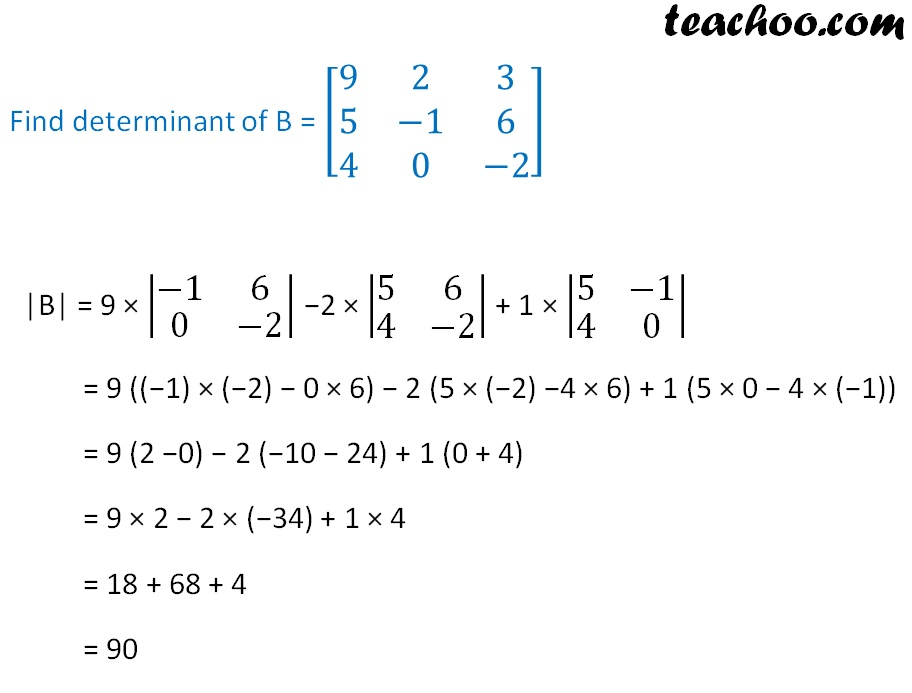## What about a 4 × 4 matrix?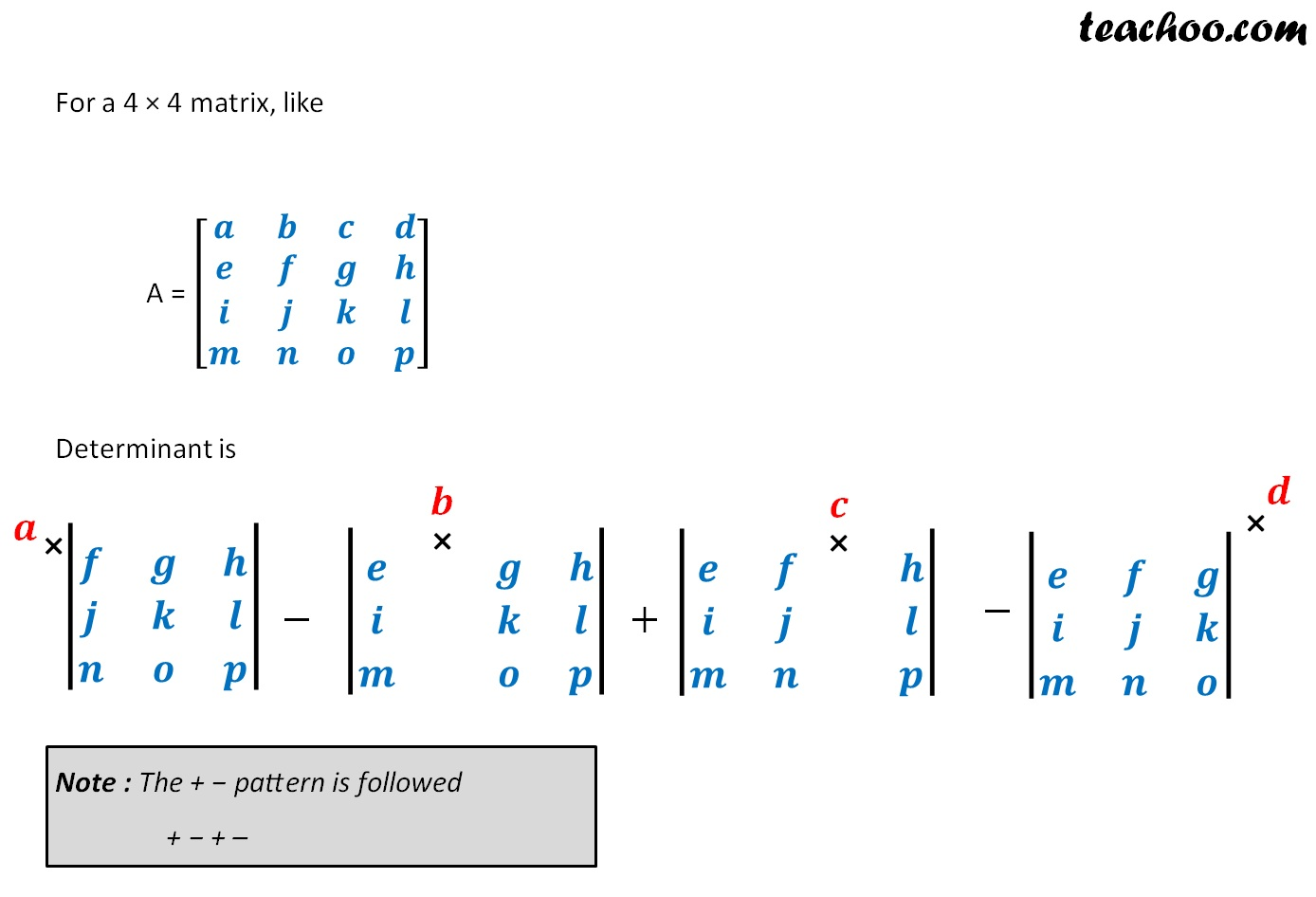Learn in your speed, with individual attention - Teachoo Maths 1-on-1 Class

### Transcript

A = [■8(𝒂&𝒃@𝒄&𝒅)] [■8(𝒂& 𝒃@𝒄& 𝒅)] Find determinant of A = [■8(3&2@1&4)] |A| = 3 × 4 - 1 × 2 = 12 − 2 = 10 For a 3 × 3 matrix, like A = [■8(𝒂&𝒃&𝒄@𝒅&𝒆&𝒇@𝒈&𝒉&𝒊)] |■8(𝒂&𝒃&𝒄@𝒅&𝒆&𝒇@𝒈&𝒉&𝒊)| = |■8( & @𝒆&𝒇@𝒉&𝒊)| − |■8( & & @𝒅& &𝒇@𝒈& &𝒊)| + |■8( & @𝒅&𝒆@𝒈&𝒉)| |A| = a (ei − hf) − b (di − gf) + c (dh − eg) Note : There is a + − pattern + − + Let’s take an example Find determinant of B = [■8(9&2&3@5&−1&6@4&0&−2)] |B| = 9 × |■8(−1&6@0&−2)| −2 × |■8(5&6@4&−2)| + 1 × |■8(5&−1@4&0)| = 9 ((−1) × (−2) − 0 × 6) − 2 (5 × (−2) −4 × 6) + 1 (5 × 0 − 4 × (−1)) = 9 (2 −0) − 2 (−10 − 24) + 1 (0 + 4) = 9 × 2 − 2 × (−34) + 1 × 4 = 18 + 68 + 4 = 90 What about a 4 × 4 matrix? For a 4 × 4 matrix, like A = [■8(𝒂&𝒃&𝒄&𝒅@𝒆&𝒇&𝒈&𝒉@𝒊&𝒋&𝒌&𝒍@𝒎&𝒏&𝒐&𝒑)] Determinant is |■8( & & @𝒇&𝒈&𝒉@𝒋&𝒌&𝒍@𝒏&𝒐&𝒑)| |■8( & & & @𝒆& &𝒈&𝒉@𝒊& &𝒌&𝒍@𝒎& &𝒐&𝒑)| |■8( & & & @𝒆& &𝒈&𝒉@𝒊& &𝒌&𝒍@𝒎& &𝒐&𝒑)| |■8( & & & @𝒆&𝒇& &𝒉@𝒊&𝒋& &𝒍@𝒎&𝒏& &𝒑)| |■8( & & @𝒆&𝒇&𝒈@𝒊&𝒋&𝒌@𝒎&𝒏&𝒐)| Note : The + − pattern is followed + − + – Matrix Matrix is representation of number in row & column format Eg: A = [■8(9&2&1@5&−1&6@4&0&−2)] Matrix can be of any order [■8(3@5@6)]_(3 × 1) [■8(3&2@1&4@5&3)]_(3 × 2) [■8(3&2@1&4)]_(2 × 2) Scalar multiplied to matrix If a number is multiplied to matrix, it is multiplied to each element of the matrix 2 [■8(9&2&1@5&−1&6@4&0&−2)] = [■8(2×9&2×2&2×1@2×5&2×(−1)&2×6@2×4&2×0&2×(−2))] Determinant Determinant is number associated with a matrix Eg: |A| = |■8(9&2&1@5&−1&6@4&0&−2)| = 90 Determinant is only possible for a square matrix |■8(3&2@1&4@5&3)| Determinant not possible |■8(3&2@1&4)| Determinant possible Scalar multiplied to determinant If a number is multiplied to determinant, it is multiplied to either one row, or one column 2 |■8(9&2&1@5&−1&6@4&0&−2)| = |■8(2×9&2×2&2×1@5&−1&6@4&0&−2)| Or |■8(2×9&2&1@2×5&−1&6@2×4&0&−2)|